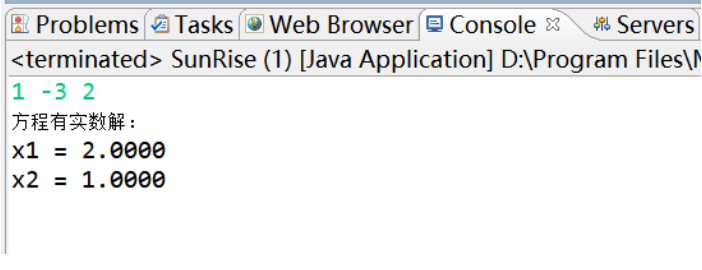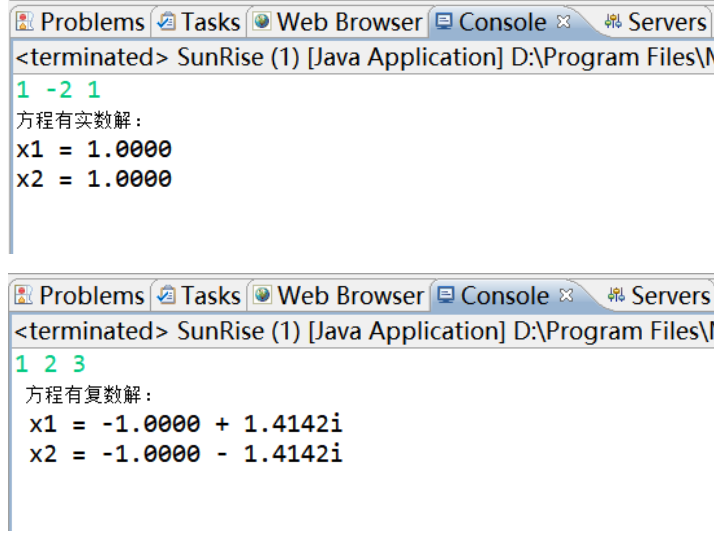• 实验目的: 1. 用MATLAB（R2008a）求方程实验原理： 二分法：
• Java求一元二次方程实验三） Package和import语句的使用 编写Java应用程序求方程。使用package语句将方程的属性即计算的方法封装在一个有包名的类中，包名是tom.jiafei，类的名字是SquareEquation。编写...
Java求一元二次方程的根（实验三）
Package和import语句的使用
编写Java应用程序求方程的根。使用package语句将方程的属性即计算根的方法封装在一个有包名的类中，包名是tom.jiafei，类的名字是SquareEquation。编写一个SunRise主类，该主类使用import语句引入tom.jiafei包中的SquareEquation类，并使用其计算方程的根。
SunRise主类
import tom.jiafei.SquareEquation;//调用另一包中的某个类
//如要调用另一个包中的所有类：import tom.jiafei.*;
public class SunRise {
public static void main(String args[]){
SquareEquation gen=new SquareEquation();
gen.Getgen();//调用方法
//		System.out.println(gen.Getgen());

}
}


SquareEquation类
package tom.jiafei;//SquareEquation类在tom.jiafei包中
import java.util.Scanner;
public class SquareEquation {
Scanner sc=new Scanner(System.in);
double a=sc.nextDouble();
double b=sc.nextDouble();
double c=sc.nextDouble();
double x1,x2,result;
public Double[] Getgen(){//返回值为一维数组（多个值）
double t=(b*b)-(4*a*c);//赋值要在方法里
if(a==0&&b==0&&c==0)
System.out.println("有无穷个解");
else if(a==0&&b==0&&c!=0)
System.out.println("方程没有解");
else if(a==0&&b!=0&&c!=0)
{
x1 =-c/b;
System.out.printf("方程有一个实数解：\n");
System.out.printf("x1 = %.4f",x1);
}
else if(t>0)
{
x1=(-b+Math.sqrt(t))/(2*a);
x2=(-b-Math.sqrt(t))/(2*a);
System.out.printf("   方程有实数解：\n");
System.out.printf("x1 = %.4f\n",x1);
System.out.printf("x2 = %.4f\n",x2);
}
else if(t==0)
{
result = -b/(2*a);
System.out.printf("方程有实数解：\n");
System.out.printf("x1 = %.4f\n",result);
System.out.printf("x2 = %.4f\n",result);
}
else if(t<0)
{
double realPart =-b/(2*a);
double imgPart =Math.sqrt(4*a*c-b*b)/(2*a);
//System.out.printf("x1 =" + realPart + "+" + imgPart + "i,  x2 = " + realPart + "-" + imgPart + "i");
System.out.printf("  方程有复数解：\n ");
System.out.printf("x1 = "+"%.4f",realPart);
System.out.printf(" + "+"%.4f",imgPart);
System.out.printf("i\n");
System.out.printf(" x2 = "+"%.4f",realPart);
System.out.printf(" - "+"%.4f",imgPart);
System.out.printf("i\n");
}
Double[] num = {x1, x2};//在没有学习用Map返回值时，可定义一维数组来返回多个平行值
//		for(int i = 0;i < num.length;i++){
//			System.out.println(num[i]);
//
//		}
return num;//小数点后保留n位可自定义
}
}

六种运行结果：展开全文• Java作业一基础语法代码 基础语法 (1) 熟悉Java程序开发...按Java Application和Applet两种方式分别编写程序，一元二次方程ax2+bx+c=0的（系数在程序中给定），并输出。 代码 package test1; import java.util.S


Java作业一
基础语法代码

基础语法
(1) 熟悉Java程序开发环境的使用 (2) 熟悉Java Application程序的结构及开发步骤 (3) 熟练掌握if语句的使用 (4) 掌握Math.sqrt()等常用数学函数的用法 (5) 熟悉System.out.print()的用法

实验内容 按Java Application和Applet两种方式分别编写程序，求一元二次方程ax2+bx+c=0的根（系数在程序中给定），并输出。
代码
package test1; import java.util.Scanner; public class count { public static void main(String[]args) { Scanner sc = new Scanner(System.in);//用Scanner类获取控制台的输入 double x1,x2; x1 = x2 = 0f; System.out.println(“当前方程表达式为：ax^2+bx+c=0”); int p; System.out.println(“请输入a,b,c的值来进行方程式的求解,输入1开始计算，输入0结束计算”); p = sc.nextInt(); if(p == 0) System.out.println(“您已结束本次计算”); while(p == 1) {
			System.out.println("请输入a的值:");//键盘输入a
double a  = sc.nextDouble();
System.out.println("请输入b的值:");//键盘输入b
double b  = sc.nextDouble();
System.out.println("请输入c的值:");//键盘输入c
double c  = sc.nextDouble();
double t;
t = b * b - 4* a * c;//判别式
if(a == 0 && b== 0)
{
System.out.println("您输入的值不能使方程式成立！请重新输入！");
}
else if(a == 0 && b != 0 && c!=0)
{
x1 = x2 = -c / b;
System.out.println("您输入的值使方程式成为一元一次方程！");
System.out.print("方程式的根为：X1 = X2");
System.out.print("x1 = ");
System.out.println(x1);
System.out.print("x2 = ");
System.out.println(x2);
}
else
{
System.out.println("您输入的值使方程式成为一元二次方程！");
if(t == 0)
{
x1 = x2 = (-b) /(2 * a);
System.out.print("方程式有两个相等实根为：X1 = X2,分别为:");
System.out.print("x1 = ");
System.out.println(x1);
System.out.print("x2 = ");
System.out.println(x2);
}
else if(t > 0)
{
x1 = ((-b) + Math.sqrt(t)) / (2 * a);
x2 = ((-b) - Math.sqrt(t)) / (2 * a);
System.out.print("方程式有两个不等实根为：X1 = X2,分别为:");
System.out.print("x1 = ");
System.out.println(x1);
System.out.print("x2 = ");
System.out.println(x2);
}
else
{
if(b != 0)
{
System.out.println("您输入的值使方程式成为一元二次方程！");
x1 = (-b) /( 2 * a);
x2 = Math.sqrt(-t) / (2 * a);
System.out.println("方程有一对共轭复根:");
System.out.print("x1 = ");
System.out.println(x1+"+"+x2+"i");
System.out.print("x2 = ");
System.out.println(x1+"-"+x2+"i");
}
else
{
x1 = Math.sqrt(-t) / (2 * a);
System.out.println("方程有一对相反虚根:");
System.out.println("x1= 0 -"+x1+"i");
System.out.println("x2= 0 +"+x1+"i");

}
}
}
System.out.println("请输入a,b,c的值来进行方程式的求解,输入1开始计算，输入0结束计算");
p = sc.nextInt();
}
}
}

展开全文• 这是数值分析课程实验中的一个实验内容——牛顿二分法求解，是用java界面实验的。
• 一元二次的方程根java,servlet,利用bean类bao装数据
• 本题目要求一元二次方程，结果保留2位小数。 输入格式: 输入在一行中给出3个浮点系数a、b、c，中间用空格分开。 输出格式: 根据系数情况，输出不同结果： 1)如果方程有两个不相等的实数，则每行输出一个，...
本题目要求一元二次方程的根，结果保留2位小数。
输入格式: 输入在一行中给出3个浮点系数a、b、c，中间用空格分开。
输出格式: 根据系数情况，输出不同结果：
1)如果方程有两个不相等的实数根，则每行输出一个根，先大后小；
2)如果方程有两个不相等复数根，则每行按照格式“实部+虚部i”输出一个根，先输出虚部为正的，后输出虚部为负的；
3)如果方程只有一个根，则直接输出此根；
4)如果系数都为0，则输出"Zero Equation"；
5)如果a和b为0，c不为0，则输出"Not An Equation"。
输入样例1: 2.1 8.9 3.5 输出样例1: -0.44 -3.80
输入样例2: 1 2 3 输出样例2: -1.00+1.41i -1.00-1.41i
输入样例3: 0 2 4 输出样例3: -2.00
输入样例4: 0 0 0 输出样例4: Zero Equation
输入样例5: 0 0 1 输出样例5: Not An Equation
代码展示
#include<stdio.h>
#include<math.h>
int main(void){
double a,b,c;
double x1,x2;
double m;
scanf("%lf %lf %lf",&a,&b,&c);
if(a==0){
if(b==0){
if(c==0){
printf("Zero Equation\n");
}else if(c!=0){
printf("Not An Equation\n");
}

} else if(b!=0){
x1=-c/b*1.0;
printf("%.2f",x1);
}
}else if(a!=0){
m=b*b-4*a*c;
if(m>0){
x1=(-b+sqrt(m))/(2.0*a);
x2=(-b-sqrt(m))/(2.0*a);
if(x1>x2){
printf("%.2f\n%.2f\n",x1,x2);
}else{
printf("%.2f\n%.2f\n",x2,x1);
}
}else if(m==0){
x1=-b/(2*a);
printf("%.2f\n",x1);
}else if(m<0){
m=-m;
double n,h;
n=-b/(2.0*a);
h=sqrt(m)/(2.0*a);
if(b!=0){
printf("%.2f+%.2fi\n%.2f-%.2fi",n,h,n,h);
}
else if(b==0){
printf("0.00+%.2fi\n0.00-%.2fi",h,h);
}
}
}
return 0;
}

ps：注意出错点会在纯虚根的printf上，即使是纯虚根输出，实部位置也要打上0.00
展开全文• 1. 一元二次方程。 2. 创建银行账号Account类（成员变量有：卡号，卡内余额等），实现对账号余额（balance）的存(deposit)、取(withdraw)和查询余额(getbalance)等功能。 3．创建一个复数类，以复数进行数学...
• 1. 编写100以内两个整数的加、减、乘、除运算测试、评分程序。 要求： 1）自动生成两个数(用文本框显示)，可选择加减乘除运算符，用户输入答案，判断对错，给出提示（用标签显示）。 2）出十道题，每题10分，测试完...
• import java.util.Scanner;//导入扫描器 public class qiufangchengdejie { public static void main(String[] args) { double a,b,c; while(true) { System.out.println(“输入a”);//实例化一个扫描器 Scanner s1=...
package mytext;
import java.util.Scanner;//导入扫描器
public class qiufangchengdejie { public static void main(String[] args) { double a,b,c; while(true) { System.out.println(“输入a”);//实例化一个扫描器 Scanner s1=new Scanner(System.in);//实例化一个扫描器 a=s1.nextDouble();//把输入的值赋给a System.out.println(“输入b”); Scanner s2=new Scanner(System.in); b=s2.nextDouble(); System.out.println(“输入c”); Scanner s3=new Scanner(System.in); c=s3.nextDouble(); if(bb-4ac<0) {//用判别式判断是否有解 System.out.println(“无解”); } else { double x1=(-b+Math.sqrt(bb-4ac))1/(2a);//利用求根公式求解 double x2=(-b-Math.sqrt(bb-4a*c))1/(2a); System.out.println(x1);//输入结果 System.out.println(x2); } }
} }
展开全文eclipse
• 今天学习了利用java求解一元二次方程。 从面向对象的角度去解决这个问题。 需要两个对象，即类 求根类（EqualRoots） 和 测试类（EqualRootsTest） 类的设计 成员方法 求根方法（EqualRoots，public） ...
• 假定在一次实际的建筑设计中，伟大的设计师经过一波辛苦的操作终于得到一个最终的函数f(x)f(x)f(x)，只需要出这个函数f(x)f(x)f(x)的零点，就可以完成整个项目的设计： y=f(x)=x2+lnx,(x>0). y=f\left(x\right)...算法 二分法
• /** * @program: shiyan1 * @Package: PACKAGE_NAME * @Description: TODO * @author: Mr.John * @date: 2020/3/19 18:54 */ public class Newton { public static void main(String[] args) { ...java
• PTA——一元二次方程 题目：本题目要求一元二次方程ax^2＋bx＋c＝0的，结果保留2位小数。 输入格式： 输入在一行中给出3个浮点系数a、b、c，中间用空格分开。 输出格式： 根据系数情况，输出不同结果： 1)...c语言
• 实验一： 使用二分法和牛顿法求方程 1．实验目的 正确理解方程求根划界法和开放法，能够编程实现其中指定的方法，并且通过比较， 分析出两类方法的优缺点。 2. 实验任务 分别用二分法和牛顿法求方程在区间[2,3]...
• 设计一个二次方程类QuadraticEquation，用于处理形如ax2 + bx + x = 0 (a≠0)的二次方程，成员如下： 私有成员a, b, c用于存储系数； 含三个参数的构造方法，用于传入a, b, c； 三个方法getA(), getB(), getC()，...
• 1. 一元二次方程。 2. 创建银行账号Account类（成员变量有：卡号，卡内余额等），实现对账号余额（balance）的存(deposit)、取(withdraw)和查询余额(getbalance)等功能。 3．创建一个复数类，以复数进行数学...
• 前言 这周一直比较忙，所以一直没有学习新的东西，所以决定本周的博客主要巩固一下课堂知识。 MVC模式 M（模型：Model）：用于储存数据的对象。 V（视图：View）：向控制器提交所...实例（计算一元二次方程） Modservlet mvc jsp
• a, b, c = map(int,input().split()) if a == 0: if b == 0: print("No") else: print('{:.2f}'.format(-c/b)) else: data = b**2 - 4*a*c if data < 0: print("No") elif data == 0: ...python
• Test类： /* (程序头部注释开始) * 程序的版权和版本声明部分 ... * 文件名称：封装一个一元二次方程根的类SquareEquation，要求考虑解方程的异常，再编写一个窗口类EquationFrame。 * 作 者： 雷恒鑫 *
• java面向对象语言的实验报告，主要是平时上课用的实验报告~
• 一、实验目的 1.设计类，并画出UML类图 2.实现UML中的类 3.使用类开发应用程序 二、实验内容 1.【矩形类Rectangle】遵照9.2节中Circle类的例子，设计一个名为Rectangle的类表示矩形。这个类包括： 两个名为width和...编程语言
• Java 语言面向对象编程技术 实验 报告 java实验
• Java2 ---实用教程最新 ------第五版 -------课后题答案
• 我写的计算方法的实验，大家不要见笑，呵呵。(江科大——费小勇)
• 即该类含有刻画方程系数的3个成员变量a,b,c以及计算实的方法getRoots， 并用数据测试该类的正确性。要求：该类的所有对象共享常数项。 如果该文章对您有些许的帮助 请多点赞关注收藏 import java.lang.Math; //...编程 对象...

# 求方程的根java实验java 订阅